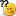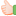# Thread: What is a output?. How will Come?.

1. ## What is a output?. How will Come?.

main()
{
int i=-1,j=-1,k=0,l=2,m;
m=i++&&j++&&k++||l++;
printf("%d %d %d %d %d",i,j,k,l,m);
}Reply With Quote

2. ## Re: What is a output?. How will Come?.

The values are i=0,j=0,k=1,l=3,m=1.its very simple here we r using post increments and logical and,or operations.Reply With Quote

3. ## Re: What is a output?. How will Come?.

I think m=0Reply With Quote

4. ## Re: What is a output?. How will Come?.

l=0,j=0,k=1,l=3,m=1;
according to relatonal operator presedence.
&& , ||,!
T&&T=T
T&&F=f
T||t=t
t||f=t
F||f=fReply With Quote

5. ## Re: What is a output?. How will Come?.

I think may be you got the error "m is not initialized before using".....

-----------------------
sureshReply With Quote

6. ## Re: What is a output?. How will Come?.

You will not get any error.
Its just application of logical operators.
Start evaluating from Left to right. All the values get post incremented.
m=i++&&j++&&k++||l++;
m = -1 && -1 && 0 || 2
since i, j, k ,l are post increments, they will increment after the complete statement evaluation.
m = 1 && 0 || 2
m = 0 || 2 , m = 1
Here, since left side is 0, it will evaluate aven the right side also.
Finally, i = 0, j = 0, k = 1, l = 3, m = 1.Reply With Quote

7. ## Re: What is a output?. How will Come?.

main()
{
int i=-1,j=-1,k=0,l=2,m;
m=i++&&j++&&k++||l++;
printf("%d %d %d %d %d",i,j,k,l,m);
}

0 0 1 3 trueReply With Quote

8. ##Re: What is a output?. How will Come?.

It will not show any errors.
The out put of your program is :
i=0;j=0;k=1;l=3;m=1(true).
m=i++&&j++&&k++||l++;

execution is as follows :
==> m= (i++&&j++)&&k++||l++; i=0 ; j=0
==> m=(-1 && -1)&&k++||l++;
==> m= (1) && k++||l++;
==> m= (1 && 0)|| l++; k= 1;
==> m= (0 || 2); ==> m= 1 l=3;

and (&&) operator truth table:

true(1) && true(1) -------> true(1)
true(1) && false(0) -------> false(0)
false(0) && true(1) -------> false(0)
false(0) && false(0) -------> true(1)

or(||) operator truth table:

true(1) || true(1) -------> true(1)
true(1) || false(0) -------> true(1)
false(0) || true(1) -------> true(1)
false(0) || false(0) -------> false(0)

::remember these while doing the logical operator programs ::

*** vishnu ***Reply With Quote

9. ## Re: What is a output?. How will Come?.Originally Posted by Goldamain()
{
int i=-1,j=-1,k=0,l=2,m;
m=i++&&j++&&k++||l++;
printf("%d %d %d %d %d",i,j,k,l,m);
}
ans:
0 0 1 3 0Reply With Quote

10. ## Re: What is a output?. How will Come?.

main()
{
int i=5;
printf("%d%d%d%d%d",i++, i--, ++i, --i,i);
}
I got the output 45545. How it will come?...Reply With Quote

11. ## Re: What is a output?. How will Come?.

ya 45545 is correct

it will check from left to right
initially i=5

so for output value i it ll print as 5

for o/p --i is a prefix it will decrease and assign value to i(5-1) so for --i its 4

for o/p ++i is a prefix it will increase and assign value to i(4+1) so for ++i its 5

for i-- is post fix so it will assign previous valuue then it will decrease by 1.

so o/p will be 5 only but after printing value will be 4

for i++ is post fix so it will assign previous valuue then it will increase by 1.

so o/p will be 4 only but after printing value will be 5

so finally value of i=5Reply With Quote

12. ## Re: What is a output?. How will Come?.

what about this?void main()
{
unsigned giveit=-1;
int gotit;
printf("%u ",++giveit);
printf("%u ",gotit=-giveit);
}Reply With Quote

13. ## Re: What is a output?. How will Come?.

hmm no idea abt unsigned intReply With Quote

14. ##Re: What is a output?. How will Come?.Originally Posted by rupesh kumar singhl=0,j=0,k=1,l=3,m=1;
according to relatonal operator presedence.
&& , ||,!
T&&T=T
T&&F=f
T||t=t
t||f=t
F||f=f
A good explaination with truth values m=1 cause at bit level
00000001 || 00000011=00000001
Nice job rupeshReply With Quote

15. ## Re: What is a output?. How will Come?.

i think u have declared giveit as unsigned and assigned "-1"Reply With Quote

16. ## Re: What is a output?. How will Come?.

Ya i declared giveit as unsigned and assigned -1 onlyReply With Quote

17. ## Re: What is a output?. How will Come?.

the o/p of this program is 00131
in given expression (m=i++&&j++&&k++||l++) operater play an imp role
i.e. we will first evaluted incre. op. that has higher predency after that we will evaluted logical AND op(&&) and after that logical OR(||) op.and at the end as.op(=) will be evaluted . note that it is a logical exp. so m have value either 1 or 0. while in this exp it is true so has 1 as o/p
and the value of i,j,k and l is incremented when write exp , so it print these incremented value by 1 so these value become 0,0,1,and 3 and the value of m is evaluted in exp ,1
dilip agrawal mca indore mpReply With Quote

18. ## Re: What is a output?. How will Come?.

m=i++ && j++ && k++ || l++;
let us leave all the postfix increments because they will change the values only in the next statement of their respective variables. so m=i && j&& k|| l
-1 && -1=0
0 && 0=0
0 || 2=1
so m is 1. this statement is over then all the variables will be incremented by 1 because of that postfix increments. so output is 00131Reply With Quote

19. ## Re: What is a output?. How will Come?.

out put vil b
-1,-1,0,2,1Reply With Quote

20. ##Re: What is a output?. How will Come?.Originally Posted by Goldamain()
{
int i=-1,j=-1,k=0,l=2,m;
m=i++&&j++&&k++||l++;
printf("%d %d %d %d %d",i,j,k,l,m);
}
i=0,j=0,k=1,l=3,m=0Reply With Quote

####Posting Permissions

• You may not post new threads
• You may not post replies
• You may not post attachments
• You may not edit your posts
•